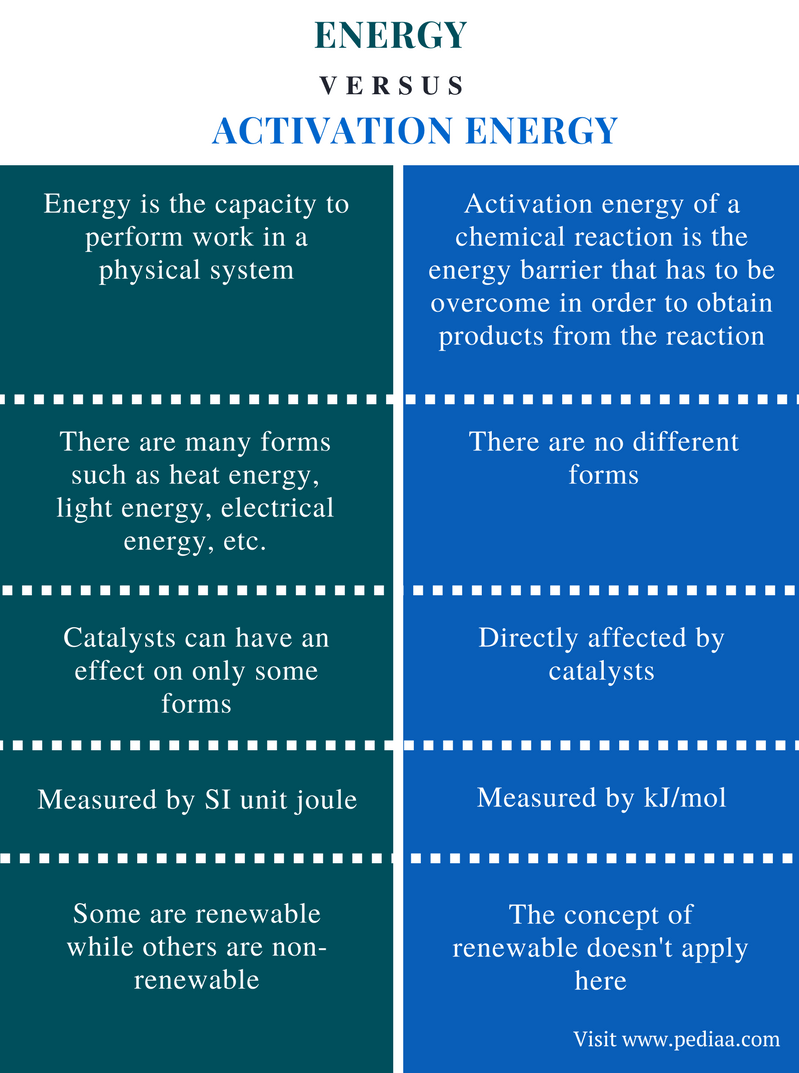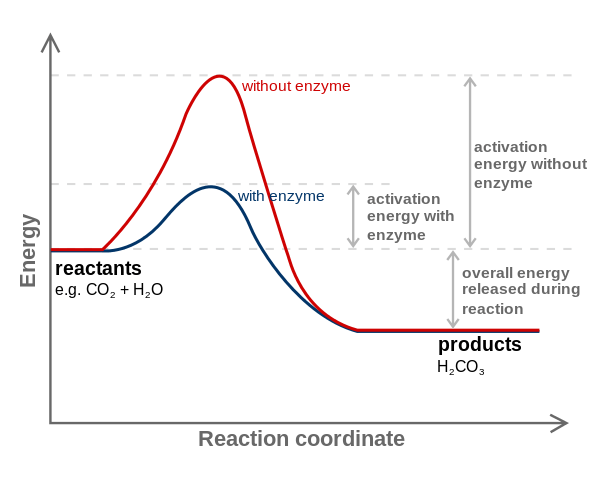# Difference Between Energy and Activation Energy

## Main Difference – Energy vs Activation Energy

Energy can be defined in different ways based on the form of energy. However, in chemistry and physics, energy is the capacity to do work. Here, “work” refers to the action of moving something against a force. Hence, energy should be given to a particular object in order to get work done. Activation energy is the minimum quantity of energy the reacting species must possess in order to undergo a specified reaction. The main difference between energy and activation energy is that energy can exist in different forms and it is the capacity to do work whereas activation energy is the energy required to form an activated complex with the highest potential energy in a chemical reaction.

### Key Areas Covered

1. What is Energy
– Definition, Different Forms, Renewable and Non-Renewable Energy, Units
2. What is Activation Energy
– Definition, Forms
3. What is the Difference Between Energy and Activation Energy
– Comparison of Key Differences

Key Terms: Activated Complex, Activation Energy, Energy, Force, Joule, Kinetic Energy, Newton, Non-Renewable, Potential Energy, Renewable, Work## What is Energy

Energy is the capacity to perform work in a physical system. “Work” refers to the action of moving something against a force. According to the law of conservation of energy, energy cannot be either created or destroyed; it can be converted from one form into a different form. There are different units used to measure energy. The SI unit is joule. One joule equals the work done on an object in order to move it 1 meter against 1 Newton force.

The total energy of a system can be either potential energy or kinetic energy. A moving system or object have both forms of energy. However, there are different forms of energy. Followings are some of the energy forms.

 Form of Energy Description Potential energy Energy stored due to the effect of gravity on the position of an object Kinetic energy Energy which a body possesses by virtue of being in motion Heat (thermal energy) Energy related to temperature Electrical energy Energy derived from the movement of charged particles Light energy A form of energy that can be seen

The forms of energy can be classified also as renewable energy and non-renewable energy. Renewable energy refers to an energy source that can be easily replenished. Non-renewable energy refers to an energy source that cannot be easily replenished.

### Renewable Energy

Following are the main forms of renewable energy.

• Solar energy
• Wind energy
• Biomass
• Hydropower
• Geothermal energy

### Non-Renewable Energy

Given below are some examples of forms of non-renewable energy.

Although the SI unit to measure energy is joule (J), there are many derived units used for the same purpose. For example, when measuring very high energies, we use kJ (kilo joule), MJ (mega joule), foot-pound force (used in the US), kilowatt-hour or kWh (for electrical energy), etc.

## What is Activation Energy

Activation energy of a chemical reaction is the energy barrier that has to be overcome in order to obtain products from the reaction. It is the minimum energy required for a reactant to convert into a product. Therefore, in order to start a chemical reaction, activation energy should be provided.

The activation energy is denoted as Ea and is measured by unit kJ/mol. The concept of activation energy is mainly used in chemistry and biochemistry. It is considered as the minimum energy required to form the intermediate with the highest potential energy in a chemical reaction. Some chemical reactions have a slow progression and occur via two or more steps. Here, intermediates are formed, which are then rearranged to form the final product. Then, the energy required to start that reaction is the energy required to form the intermediate with highest potential energy. Activation energy is also considered as an energy barrier.Figure 2: Effect of Enzymes on Activation Energy

Catalysts can reduce the activation energy. Therefore, catalysts are often used in order to overcome the energy barrier and let the chemical reaction to progress. Enzymes are biological catalysts. They can reduce the activation energy of reaction take place in tissues. The above image gives one such example.

## Difference Between Energy and Activation Energy

### Definition

Energy: Energy is the capacity to perform work in a physical system.

Activation Energy: Activation energy of a chemical reaction is the energy barrier that has to be overcome in order to obtain products from the reaction.

### Different Forms

Energy: There are many forms of energy such as heat energy, light energy, electrical energy, etc.

Activation Energy: There are no different forms of activation energy.

### Effect of Catalysts

Energy: Catalysts do not have an effect on all forms of energy but on some forms such as activation energy.

Activation Energy: Activation energy is directly affected by catalysts. Catalysts can reduce the activation energy.

### Units

Energy: Energy is measured by SI unit joule.

Activation Energy: Activation energy is measured by kJ/mol.

### Renewability

Energy: Some energy forms are renewable while others are non-renewable.

Activation Energy: There is no renewability regarding activation energy.

### Conclusion

Activation energy is a form of energy. The main difference between energy and activation energy is that energy can exist in different forms and it is the capacity to do work whereas activation energy is the energy required to form the activated complex with the highest potential energy in a chemical reaction.

##### References:

1. “What Is Energy? – Energy Explained, Your Guide To Understanding Energy .”Energy Information Administration, Available here.
2. Jones, Andrew Zimmerman. “Energy: A Scientific Definition.” ThoughtCo, Aug. 10, 2017, Available here.
3. Helmenstine, Anne Marie, Ph.D. “Activation Energy Definition – Ea in Chemistry.” ThoughtCo, Apr. 20, 2017, Available here.

##### Image Courtesy:

1. “1689721” (Public Domain) via Pixabay
2. “Carbonic anhydrase reaction in tissue” By Fvasconcellos (talk · contribs) – Image:Activation2 updated.svg (CC BY-SA 3.0) via Commons Wikimedia